# Numerical Methods for Economists: The Solow (1956) Model¶

Welcome to the first official Numerical Methods for Economists lab session! This focus of this lab session will be solving and simulating the Solow model of economic growth from Chapter 1 of Romer's Advanced Macroeconomics. Although Romer's treatment is excellent, I highly recommend reading Solow's original journal article.

Here is a quick summary of what we will cover today:

• Task 1: Coding the Solow model in Python.
• Task 2: Calibrating the model using data from the Penn World Tables.
• Task 3: Graphical analysis of the Solow model using Matplotlib.
• Task 4: Assessing the stability of the Solow model.
• Task 5: Simulating the Solow model.
• Task 6: Finite-difference methods for solving initial value problems.
• Task 7: Piece-wise linear interpolation.

## Task 1: Coding the Solow model in Python¶

In this task you will learn how to program a continuous time version of the Solow model in Python. Before we starting writing code, we should stop and think about the "primitives" (i.e., basic bulding blocks) of a Solow model. Writing code that uses the only the most basic bulding blocks will allows us to use the same code to solve and simulate as a general a model as possible.

The classic Solow growth model is completely described by the equation of motion for capital (per person/effective person)

\begin{equation} \dot{k} = sf(k(t)) - (n + g + \delta)k(t). \tag{1.1} \end{equation}

Thus in order to construct the equation of motion for capital, we need only specify a functional form for $f$ and provide some values for the parameters $s,\ n,\ g,\ \delta,\ \alpha$. A common functional form for technology is the constant elasticity of substitution (CES) production function

\begin{equation} Y(t) = \bigg[\alpha K(t)^{\rho} + (1-\alpha) (A(t)L(t))^{\rho}\bigg]^{\frac{1}{\rho}} \tag{1.2} \end{equation}

where $0 < \alpha < 1$ and $-\infty < \rho < 1$. The parameter $\rho = \frac{\sigma - 1}{\sigma}$ where $\sigma$ is the elasticity of substitution between factors of production. The CES production technology is popular because it nests several interesing special cases. In particular, if factors of production are perfect substitutes (i.e., $\sigma = +\infty \implies \rho = 1$), then output is just a linear combination of the inputs.

\begin{equation} \lim_{\rho \rightarrow 1} Y(t) = \alpha K(t) + (1-\alpha)A(t)L(t) \tag{1.3} \end{equation}

On the other hand, if factors of production are perfect complements (i.e., $\sigma = 0 \implies \rho = -\infty$), then we recover the Leontief production function.

\begin{equation} \lim_{\rho \rightarrow -\infty} Y(t) = \min\left\{\alpha K(t), (1-\alpha) A(t)L(t)\right\} \tag{1.4} \end{equation}

Finally, if the elasticity of substitution is unitary (i.e., $\sigma=1 \implies \rho=0$), then output is Cobb-Douglas.

\begin{equation} \lim_{\rho \rightarrow 0} Y(t) = K(t)^{\alpha}(A(t)L(t))^{1-\alpha} \tag{1.5} \end{equation}

For the remainder of the lab we will work with the intensive form of the Cobb-Douglas prodution technology.

\begin{equation} y(t) = \frac{Y(t)}{A(t)L(t)} = f(k) = k(t)^{\alpha} \tag{1.6} \end{equation}

Now that we have chosen our functional form for production, we are ready to code the Solow model. We being with some standard import statements:

• numpy: The foundation upon which all scientific computing is Python is built.
• pandas: Kick-ass tool for data analysis in Python. Pandas combined with statsmodels can do most all of your econometrics. Pandas is quickly becoming a standard tool for analysis of financial data.
• scipy: SciPy is open-source software for mathematics, science, and engineering. It is also the name of a very popular conference on scientific programming with Python. SciPy is built on top of NumPy.
• matplotlib: Primary graphics engine for Python.
• growth: A Python module for solving and simulating continuous-time growth models.
In :
import numpy as np
import pandas as pd
from scipy import integrate, interpolate, linalg, optimize
import matplotlib as mpl
import matplotlib.pyplot as plt

# for the first few labs we will be working with models of growth
import growth
import pwt


### Coding $f(k)$ and $f'(k)$ in Python:¶

Once we have settled on the Cobb-Douglas functional form for production, we need to write Python functions which encode the intensive form of the Cobb-Douglas production technology and the marginal product of capital.

In :
def cobb_douglas_output(t, k, params):
"""
Cobb-Douglas production function.

Arguments:

t:      (array-like) Time.
k:      (array-like) Capital (per person/effective person).
params: (dict) Dictionary of parameter values.

Returns:

y: (array-like) Output (per person/ effective person)

"""
# extract params
alpha = params['alpha']

# Cobb-Douglas production function
y = k**alpha

return y

def marginal_product_capital(t, k, params):
"""
Marginal product of capital with Cobb-Douglas production function.

Arguments:

t:      (array-like) Time.
k:      (array-like) Capital (per person/effective person).
params: (dict) Dictionary of parameter values.

Returns:

y_k: (array-like) Derivative of output with respect to capital, k.

"""
# extract params
alpha = params['alpha']

# marginal product of capital
mpk = alpha * k**(alpha - 1)

return mpk


Quick note about docstrings and their importance: Python documentation strings (or docstrings) provide a convenient way of associating documentation with Python modules, functions, classes, and methods. An object's docsting is defined by including a string constant as the first statement in the object's definition. Writing good docstrings for functions is an important part of of doing reproducible research. Never write a function without a docstring!

In :
# we can inspect a functions docstring using a question mark...
cobb_douglas_output?


### Coding $\dot{k}$ in Python:¶

Next we need to write another Python function encoding the equation of motion for capital per effective person.

In :
def equation_of_motion_capital(t, k, params):
"""
Equation of motion for capital (per person/effective person).

Arguments:

t:      (array-like) Time.
k:      (array-like) Capital (per person/effective person).
params: (dict) Dictionary of parameter values.

Returns:

k_dot: (array-like) Time-derivative of capital (per person/effective
person).

"""
# extract params
s     = params['s']
n     = params['n']
g     = params['g']
delta = params['delta']

# equation of motion for capital
k_dot = s * cobb_douglas_output(t, k, params) - (n + g + delta) * k

return k_dot


### Coding $\frac{\partial\dot{k}}{\partial k}$ in Python:¶

The final piece of the Solow model is a Python function returning the value of the derivative of the equation of motion for capital (per worker/effective worker) with respect to $k$ (i.e., the Jacobian of the Solow model).

In :
def solow_jacobian(t, k, params):
"""
The Jacobian of the Solow model.

Arguments:

t:      (array-like) Time.
k:      (array-like) Capital (per person/effective person).
params: (dict) Dictionary of parameter values.

Returns:

jac: (array-like) Value of the derivative of the equation of
motion for capital (per person/effective person) with
respect to k.

"""
# extract params
s     = params['s']
n     = params['n']
g     = params['g']
delta = params['delta']

k_dot = s * marginal_product_capital(t, k, params) - (n + g + delta)

return k_dot


Now that we have our model coded, we need to define some parameter values. Recall that the of exogenous parameters of the Solow Model with Cobb-Douglas production are...

• $s$: savings rate
• $n$: labor force growth rate
• $g$: growth rate of technology
• $\alpha$: capital share of output
• $\delta$: rate of capital depreciation
• $L(0)$: Initial value for labor supply.
• $A(0)$: Initial level of technology.

To store the values of the exogenous parameters of the Solow model we will create a Python dictionary. Where do we get these parameter values? Common method is to pull "sensible" values out of ones arse. A better method, which we will cover in the nest task, would be to calibrate the values of the parameters based on real world data.

In :
# sensible parameter values for the Solow model
solow_params = {'alpha':0.33, 'delta':0.04, 'n':0.01, 'g':0.02, 's':0.15, 'A0':1.0, 'L0':1.0}


Now we are ready to create an instance of the SolowModel Python class which will represent our Solow model!

In :
# inspect the SolowModel object...
growth.SolowModel?

In :
# create an instance of the SolowModel class representing our model!
solow = growth.SolowModel(output=cobb_douglas_output,
mpk=marginal_product_capital,
k_dot=equation_of_motion_capital,
jacobian=solow_jacobian,
params=solow_params)


### Coding the steady state value of $k$ in Python¶

When analyzing growth models we are often care about the long-run steady state of the model. Because the Solow Model is so simple, we can write down an analytic expression for the steady state value of capital (per worker/effective worker) in terms of the structural parameters of the model.

\begin{equation} k^* = \left(\frac{s}{n+g+\delta}\right)^{\frac{1}{1-\alpha}} \tag{1.7} \end{equation}

We code this expression as a Python function as follows...

In :
def analytic_k_star(params):
"""Steady-state level of capital (per person/effective person)."""
# extract params
s     = params['s']
n     = params['n']
g     = params['g']
alpha = params['alpha']
delta = params['delta']

return (s / (n + g + delta))**(1 / (1 - alpha))


..and then we add this expression to our SolowModel instance by first creating a Python dictionary containing the steady state expression and adding it to the model.

In :
# create a dictionary of steady state expressions...

In :
# add the dictionary of functions to the model


In this task we a simple strategy for calibrating a Solow model with Cobb-Douglas production using data from the Penn World Tables (PWT).

### Capital depreciation rate, $\delta$:¶

The PWT provides estimated depreciation rates that vary across both time and countries. As an estimate for the rate of capital depreciation for country $i$ I use the time-average of $\verb|delta_k|_{it}$ as provided by the PWT.

### Capital's share of output/income, $\alpha$:¶

Capital's share for country $i$ in year $t$, $\alpha_{it}$ is computed as $\alpha_{it} = 1 - \verb|labsh|_{it}$, where $\verb|labsh|_{it}$ is the labor share of output/income for country $i$ in year $t$ provided by the PWT. I then use the time-average of $\alpha_{it}$ as the estimate of capital's share for country $i$.

### Savings rate, $s$:¶

As a measure of the savings rate for country $i$, I take the simple time-average of the annual investment share of real GDP, $\verb|i_sh|$, for country $i$ from the PWT.

### Labor force growth rate, $n$:¶

To obtain a measure of the labor force growth rate for country $i$, I regress the logarithm of total employed persons, $\verb|emp|$, in country $i$ from the PWT on a constant and a linear time trend.

$$\ln\ \verb|emp|_i = \beta_0 + \beta_1 \verb|t| + \epsilon_i \tag{2.1}$$

The estimated coefficient $\beta_1$ is then used as my estimate for the $n$. To estimate the initial number of employed persons, $L_0$, I use $e^{\beta_0}$ as the estimate for $L_0$.

### Growth rate of technology, $g$:¶

To obtain a measure of the growth rate of technology for country $i$, I first adjust the total factor productivity measure reported by the PWT, $\verb|rtfpna|$ (which excludes the human capital contribution to TFP), and then regress the logarithm of this adjusted measure of TFP, $\verb|atfpna|$, for country $i$ on a constant and a linear time trend.

$$\ln\ \verb|atfpna|_i = \beta_0 + \beta_1 \verb|t| + \epsilon_i \tag{2.2}$$

The estimated coefficient $\beta_1$ is then used as my estimate for the $g$. To estimate the initial level of technology, $A_0$, I use $e^{\beta_0}$ as the estimate for $A_0$.

All of this is being done "behind the scenes". All you need to in order to calibrate the model is pick an ISO 3 country code! Now let's calibrate a Solow model for the UK.

In :
# calibrate the Solow model for GBR
growth.calibrate_cobb_douglas(model=solow, iso3_code='GBR')

# display the results
solow.params

Out:
{'A0': 0.94757946906339341,
'L0': 22.66865316643656,
'alpha': 0.36286396,
'delta': 0.041320667,
'g': 0.018602292580074467,
'n': 0.0036491168322516018,
's': 0.18750289,
'sigma': 1.0}
In :
# display k_star for our chosen parameter values
print 'k* =', analytic_k_star(solow.params)

k* = 5.46097822832

In :
# compare with our model's dictionary of steady state values

Out:
{'k_star': 5.4609782283190977}

### Short digression about numerical precision:¶

The equation of motion for capital (per person/effective person) should return zero when evaluated at the steady state, $k^*$. Let's check whether or not it does...

In :
# very close, but not exactly zero!
print 'capital(k*) =', solow.k_dot(0, analytic_k_star(solow.params), solow.params)

capital(k*) = 0.0


To a human this is almost, but not "exactly", zero. But for most computers there is no difference between this number and exactly zero! Why? No computer has infinite precision. Eventually, at a small enough resolution, a computer can no longer tell the difference between two floating point numbers. My computer, which is a 32-bit machine, has the following machine-$\epsilon$ (i.e., machine "epsilon").

In :
# this is your machine epsilon
np.finfo('float').eps

Out:
2.2204460492503131e-16

If you have a 64-bit computer, then your machine-$\epsilon$ will be even smaller!.

### Exercise 1:¶

#### Part a)¶

Derive analytical expressions for the deterministic steady state values of output per effective worker, $y$, and consumption per effective worker, $c$, and then use your results to fill in the blanks in the code below.

In [ ]:
def analytic_y_star(params):
"""The steady-state level of output per effective worker."""
# extract params
s     = params['s']
n     = params['n']
g     = params['g']
alpha = params['alpha']
delta = params['delta']

y_star = # insert your code here!

return y_star

def analytic_c_star(params):
"""The steady-state level of consumption per effective worker."""
# extract params
s     = params['s']
n     = params['n']
g     = params['g']
alpha = params['alpha']
delta = params['delta']

c_star = # insert your code here!

return c_star


#### Part b)¶

Create a new dictionary called new_steady_state_funcs containing the expression for $k^*, y^*,$ and $c^*$.

In [ ]:
# insert your code here!


#### Part c)¶

Use the set_functions method of the SolowModel class's steady_state attribute to add the new dictionary of steady state functions to your model. Then use the set_values method of the steady_state attribute to re-compute the steady state values.

In [ ]:
# insert your code here!


#### Part d)¶

Examine the resulting dictionary which should now contain three values (one for $k^*, y^*$ and $c^*$).

In [ ]:
# insert your code here!


### Exercise 2:¶

Try calibrating your model and computing the steady state values for at least 5 different countries. How different are the steady state values across countries? Are there any groups of countries that you think might have systematically lower/higher steady state values?

In [ ]:
# insert your code here!


## Task 3: Graphical analysis of the Solow model using Matplotlib¶

In this task you will learn how to recreate some of the basic diagrams used to analyze the Solow model using the Python library Matplotlib. We start by changing different parameters to see how each change impacts the long-run steady state of the model. We then focus on short-run dynamics by plotting impulse response functions for various parameter changes.

### Long-run comparative statics¶

In :
# set up inline plotting!
%matplotlib inline

In :
# create a new figure
fig = plt.figure(figsize=(8,6))

# create a Solow Diagram in one line!
solow.plot_solow_diagram(gridmax=15)

# Save and display the figure
plt.savefig('graphics/Classic-Solow-Diagram.png')
plt.show()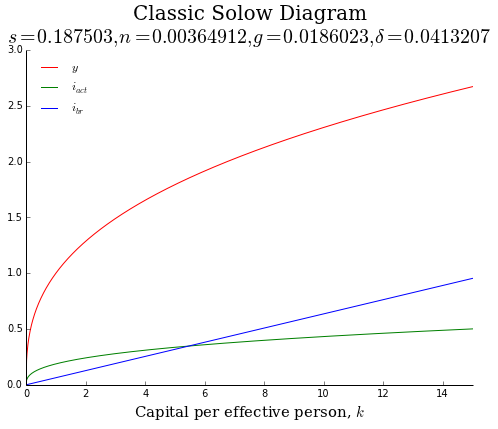Suppose that we want to change parameter values of the model in order to see how the Solow diagram changes. The code in the cell below analyzes a doubling of the savings rate.

In :
# create a new figure
fig = plt.figure(figsize=(8,6))

# plot comparative statics for the solow model using one line of code!
solow.plot_solow_diagram(gridmax=50, param='s', shock=2.0, reset=True)

# display the figure
plt.show()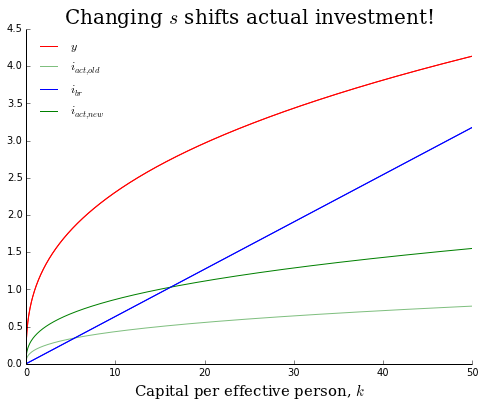### Exercise 3:¶

Describe how, if at all, each of the following developments affects the break-even and actual-investment lines in our basic diagram for the Solow model:

#### Part a)¶

The rate of depreciation, $\delta$, falls by 10%.

In [ ]:
# insert your code here!

In :
# my solution for GBR
%run exercise_3a.py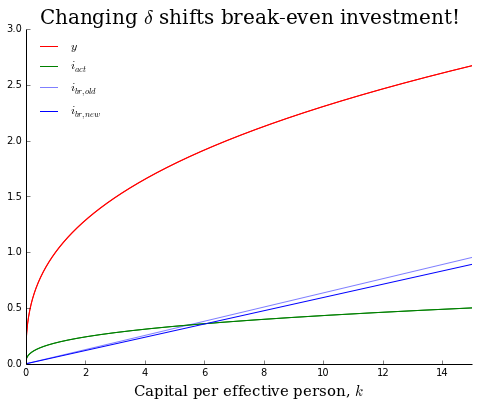#### Part b)¶

The rate of technology growth, $g$, doubles.

In [ ]:
# insert your code here!

In :
# my solution for GBR
%run exercise_3b.py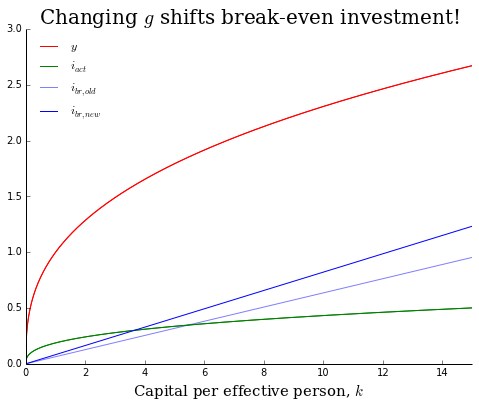#### Part c)¶

Capital's share of income/output, $\alpha$, increases by 50%.

In [ ]:
# insert your code here!

In :
# my solution for GBR
%run exercise_3c.py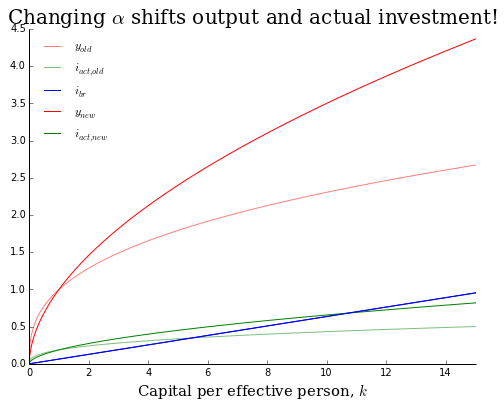### Short-run comparative statics¶

Suppose that we start the Solow economy in steady-state with current parameter values and then at $t=2000$ shock the economy by doubling the savings rate. How should we expect the time-paths of captial, output, and consumption per effective worker to respond?

We can assess the short-run comparative statics of the Solow model by plotting impulse response functions (IRFs). The plot_impulse_response method of the SolowModel class can be used to plot three different types of impulse response functions (i.e., efficiency_units, per_capita, or levels) following a multiplicative shock to any of the models exogenous parameters. The syntax for plotting the IRF for a doubling of the savings rate in efficiency units would be as follows...

In :
# calibrate for the ZWE
growth.calibrate_cobb_douglas(model=solow, iso3_code='ZWE')

# plot the impulse response function
fig_kwargs = {'figsize':(12,8)}
solow.plot_impulse_response(variables='all',         # which variables to plot? 'all', 'k', 'y', 'c'?
param='s',               # parameter we wish to shock
shock=1.5,               # magnitude of the multiplicative shock
T=100,                   # length of the IRF
color='b',               # color for the irfs
year=1970,               # year in which the shock hits
kind='efficiency_units', # what type of IRFs to plot
log=False,               # logarithmic scale for y-axis?
reset=True,              # reset parameter values following shock?
**fig_kwargs)
plt.show()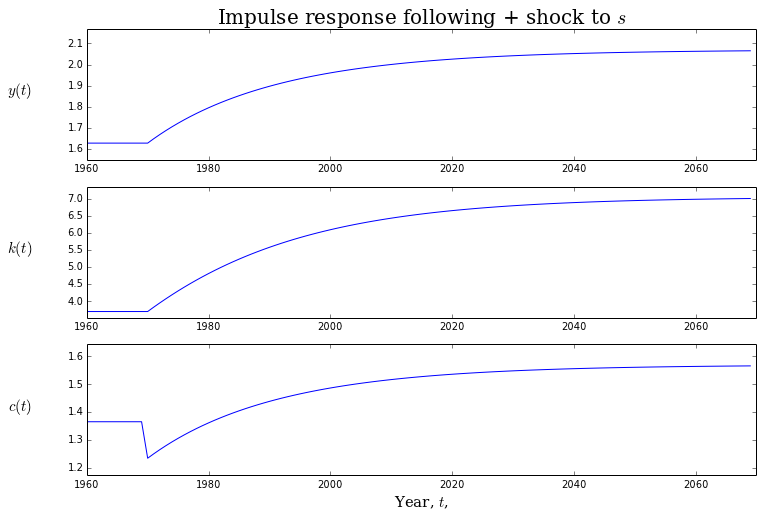Increasing the savings rate will cause both capital and output per effective worker to increase montonically towards their new, higher long-run steady state values. In the short-run, consumption per effective worker must fall; however, the long-run impact on consumption per effective worker is ambiguous and depends on the position of the ex ante and ex post steady state levels of capital per effecetive worker relative to the "Golden-rule" level and the magnitude of the shock.

### Exercise 4:¶

Plot impulse response functions for the following parameter changes in efficiency units, per capita units, and levels by changing the kind argument of the plot_impulse_response method to kind=='efficiency_units', kind=='per_capita', kind=='levels', respectively.

#### Part a)¶

A positive shock to capital's share, $\alpha$.

In [ ]:
# insert your code here!

In :
# my solution for GBR in efficiency units
%run exercise_4a.py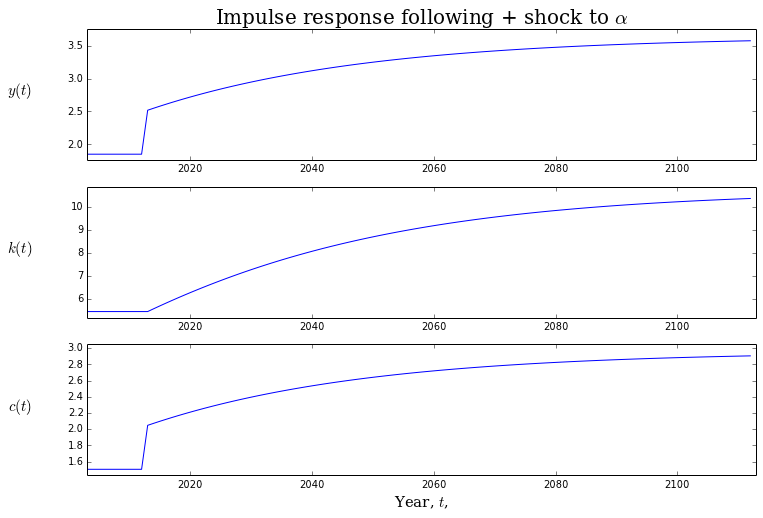#### Part b)¶

A negative shock to the population growth rate, $n$.

In [ ]:
# insert your code here!

In :
# my solution for GBR in per capita units
%run exercise_4b.py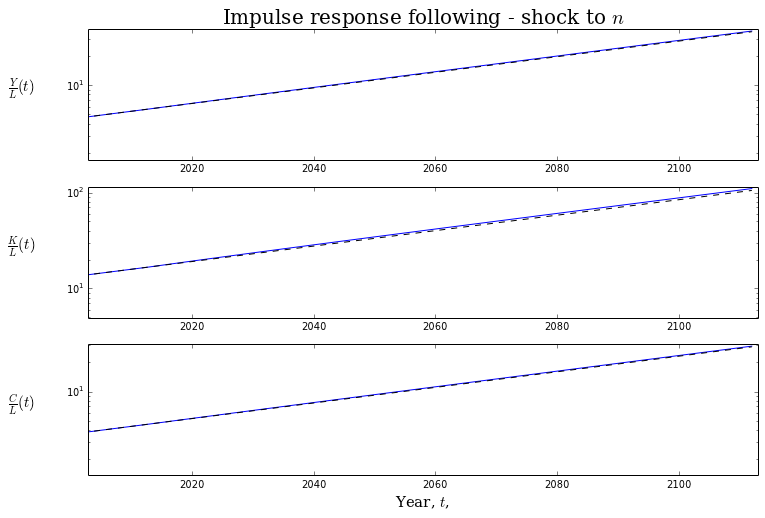#### Part c)¶

A negative shock to the growth rate of technology, $g$.

In [ ]:
# insert your code here!

In :
# my solution for GBR in per capita units
%run exercise_4c.py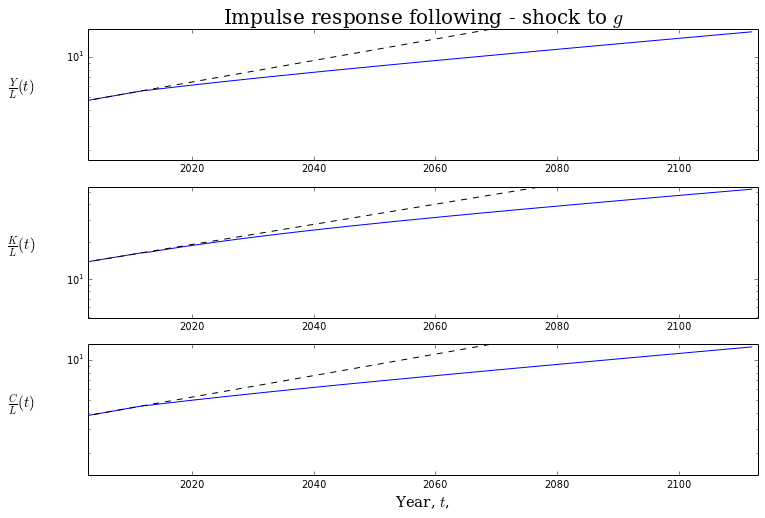#### Part d)¶

A positive shock to the capital depreciation rate, $\delta$.

In [ ]:
# insert your code here!

In :
# my solution for GBR in efficiency units
%run exercise_4d.py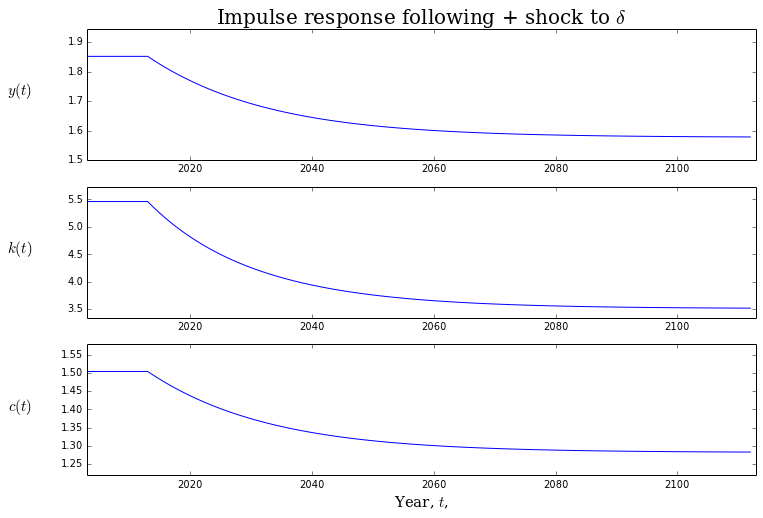#### Part e)¶

A negative shock to the savings rate, $s$.

In [ ]:
# insert your code here!

In :
# my solution for GBR in efficiency units
%run exercise_4e.py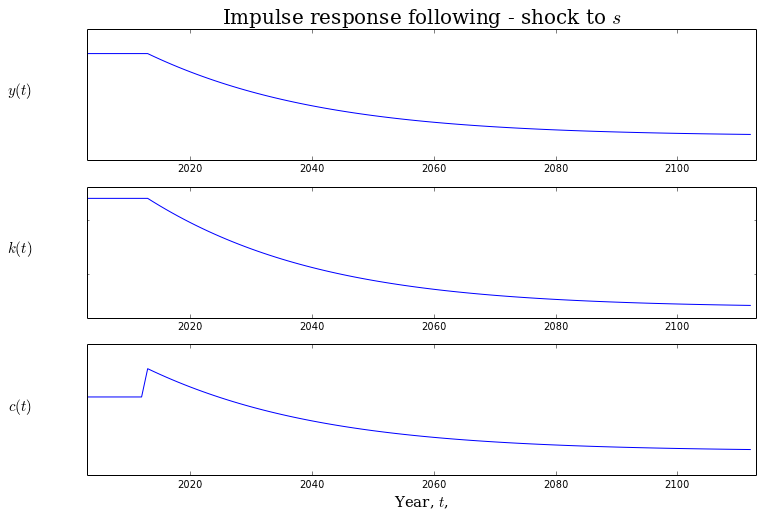## Task 4: Assessing the stability of the Solow Model¶

So long as the initial levels of capital, labor, and technology are all strictly positive, the Solow model is globally stable: given any initial condition for capital per effective worker, $k$, the dynamics of the Solow model will push $k$ towards its long-run, steady state value, $k^*$. This information can be summarized graphically by a phase diagram. The SolowModel class has a method for plotting the phase diagram of the model...

In :
# calibrate the model to Germany
growth.calibrate_cobb_douglas(model=solow, iso3_code='DEU')

In :
# compare to figure 1.3 from Chapter 1 of Romer's text!
fig = plt.figure(figsize=(8,6))
solow.plot_phase_diagram()
plt.show()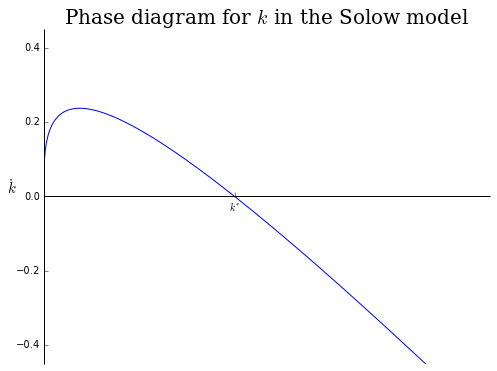The phase diagram suggests another way to assess stability of the Solow model: the derivative of the equation of motion for capital per effective worker should be negative when evaluated at the steady state value, $k^*$.

### Exercise 5:¶

Evaluate the Jacobian for the Solow model at the steady state value of capital per effective worker and confirm that the result is indeed negative.

In [ ]:
# insert your code here!


We can overlay a plot of the linearized equation of motion for capital (per person/effective person) for comparison purposes. For values of $k < k^*$, the linearized equation of motion will lead to an over-estimate of the true speeed of convergence; for value of $k > k^*$, the lineaized equation of motion will lead to an under estimate of the true speed of convergence.

In :
fig = plt.figure(figsize=(8,6))
solow.plot_phase_diagram(linearize=True)
plt.show()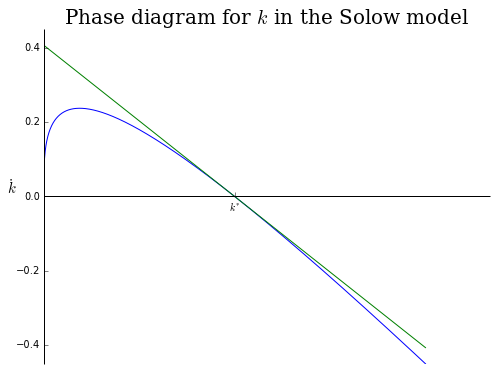For the Germany, the speed of convergence computed using the linearized equation of motion over estimates the true speed of convergence implied by the model by roughly 1.1%.

In :
k_star = solow.steady_state.values['k_star']
grid   = np.linspace(0.5 * k_star, 1.5 * k_star, 1000)

# percentage approximation error for speed of convergence...
percentage_error = (solow.k_dot(0, grid, solow.params) - solow.linearized_k_dot(grid)) / solow.k_dot(0, grid, solow.params)

In :
# average error
np.mean(percentage_error[np.isfinite(percentage_error)])

Out:
-0.010917816468389819

## Task 5: Simulating the Solow Model¶

Before discussing these numerical methods we should stop and consider whether or not there are any special cases (i.e., combintions of parameters) for which the Solow model might have an analytic solution. Analytic results can be very useful in building intuition about the economic mechanisms at play in a model and they are invaluable for debugging code and assessing the accurayc of numerical methods.

It just so happens that the Solow model with Cobb-Douglas production has a general analytic solution!

### Analytic solution¶

The Solow model with Cobb-Douglas production happens to have a completely general analytic solution (see handout for the mathematics if interested in how this result is derived).

$$k(t) = \left[\left(\frac{s}{n+g+\delta}\right)\left(1 - e^{-(n + g + \delta) (1-\alpha) t}\right) + k_0^{1-\alpha}e^{-(n + g + \delta) (1-\alpha) t}\right]^{\frac{1}{1-\alpha}}$$

Here is a Python function encoding the analytic solution...

In :
def solow_analytic_solution(k0, t, params):
"""
Computes the analytic solution for the Solow model with Cobb-Douglas
production technology.

Arguments:

k0:     (float) Initial value for capital (per person/effective person)

t:      (array-like) (T,) array of points at which the solution is
desired.

params: (dict) Dictionary of model parameters.

Returns:

analytic_traj: (array-like) (T,2) array representing the analytic
solution trajectory.

"""
# extract parameter values
g     = params['g']
n     = params['n']
s     = params['s']
alpha = params['alpha']
delta = params['delta']

# lambda governs the speed of convergence
lmbda = (n + g + delta) * (1 - alpha)

# analytic solution for Solow model at time t
k_t   = (((s / (n + g + delta)) * (1 - np.exp(-lmbda * t)) +
k0**(1 - alpha) * np.exp(-lmbda * t))**(1 / (1 - alpha)))

# combine into a (T, 2) array
analytic_traj = np.hstack((t[:,np.newaxis], k_t[:,np.newaxis]))

return analytic_traj


We can generate an analytic trajectory for our Solow model like so...

In :
# specify some initial conditions

# grid of t values for which we want to value of k(t)
grid = np.linspace(0, 100, 10)

# generate a trajectory!
solow_analytic_solution(k0, grid, solow.params)

Out:
array([[   0.        ,    5.34659456],
[  11.11111111,    7.05381168],
[  22.22222222,    8.25264562],
[  33.33333333,    9.07063436],
[  44.44444444,    9.62009231],
[  55.55555556,    9.98579925],
[  66.66666667,   10.22784247],
[  77.77777778,   10.38747556],
[  88.88888889,   10.49252128],
[ 100.        ,   10.56154632]])

...and we can make a plot of this trajectory like so...

In :
plt.figure(figsize=(8,6))

# plot this trajectory
analytic_trajectory = solow_analytic_solution(k0, grid, solow.params)
plt.plot(grid, analytic_trajectory[:,1], 'r-')

# demarcate k*
color='k', label='$k^*$')

# axes, labels, title, etc
plt.xlabel('Time, $t$', fontsize=15)
plt.ylabel('$k(t)$', rotation='horizontal', fontsize=15, family='serif')
plt.title('Analytic trajectory for the Solow model', fontsize=20, family='serif')
plt.legend(loc='best', frameon=False)

plt.show()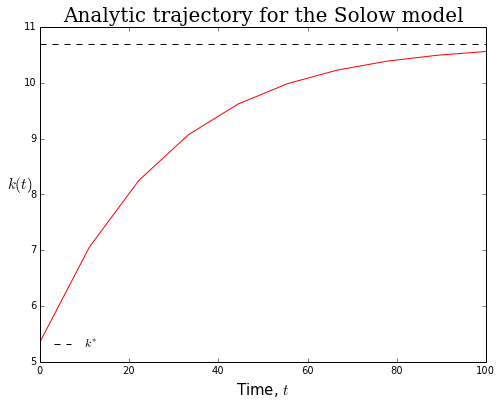...finally, let's plot trajectories for different initial conditions.

In :
plt.figure(figsize=(8,6))

# lower and upper bounds for initial conditions

for k0 in np.linspace(k_l, k_u, 5):
# plot this trajectory
plt.plot(grid, solow_analytic_solution(k0, grid, solow.params)[:,1])

# demarcate k*
color='k', label='$k^*$')

# axes, labels, title, etc
plt.xlabel('Time, $t$', fontsize=15, family='serif')
plt.ylabel('$k(t)$', rotation='horizontal', fontsize=15, family='serif')
plt.title('Analytic trajectories for the Solow model', fontsize=20, family='serif')
plt.legend(loc='best', frameon=False)

plt.show()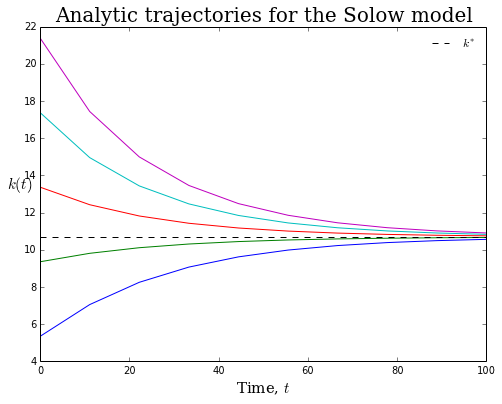### Linearized solution:¶

As discussed in your lectures, a commonly used approach to study the behavior of the Solow model is to linearize the equation of motion for capital (per person/effective person) around its long-run equilibrium value of $k^*$.

The code in the cell below computes analytic and linearized solution trajectories and then plots them for comparison.

In :
plt.figure(figsize=(8,6))

# plot analytic and linearized trajectoryies
grid = np.linspace(0, 100, 100)

analytic_trajectory = solow_analytic_solution(k0, grid, solow.params)
linearized_trajectory = solow.linearized_solution(k0, grid)

plt.plot(grid, analytic_trajectory[:,1], 'r-', label='Analytic')
plt.plot(grid, linearized_trajectory[:,1], 'b-', label='Linearized')

# demarcate k*
color='k', label='$k^*$')

# axes, labels, title, etc
plt.xlabel('Time, $t$', fontsize=15)
plt.ylabel('$k(t)$', rotation='horizontal', fontsize=15, family='serif')
plt.title('Analytic trajectory for the Solow model', fontsize=20, family='serif')
plt.legend(loc='best', frameon=False, prop={'family':'serif'})

plt.show()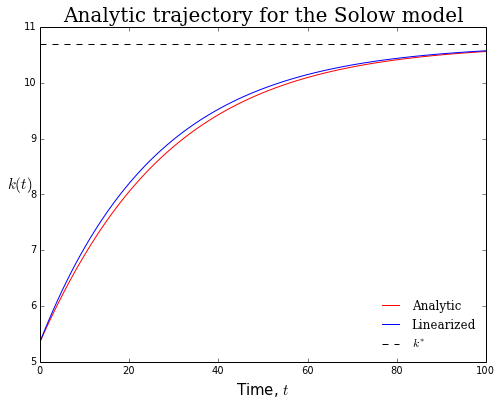Compute the approximation error of the linearized solution using the $L^2$ norm (i.e., euclidean distance).

$$L^2 \text{error} = \left[\sum_{i=0}^n(traj_{1,i} - traj_{2,i})^2\right]^{\frac{1}{2}}$$
In [ ]:
# inspect the source code for the get_L2_errors method
solow.get_L2_errors??

In :
# compute the L2 errors
solow.get_L2_errors(analytic_trajectory, linearized_trajectory)

Out:
0.86541144604327747

Alternative way of computing the approximation error of the linearized solution using the $L^{\infty}$ norm (i.e., maximal distance).

$$L^{\infty} \text{error} = \max_{i=1,\dots,n} |traj_{1,i} - traj_{2,i}|$$
In :
# compute the maximal errors
solow.get_maximal_errors(analytic_trajectory, linearized_trajectory)

Out:
0.14817898066327295

Although the $L^2$ and $L^{\infty}$ metrics are often used to measure approximation error, it is often not obvious how to interpret their magnitudes. Another measure, that is often more interpretable (if less formal), is to simply normalize the absolute differences between the two trajectories by the analytic solution and take the average.

In :
# absolute percentage errors
np.mean(solow.compare_trajectories(analytic_trajectory, linearized_trajectory)[:,0] / analytic_trajectory[:,1])

Out:
0.0085749847823672325

In general, the absolute magnitude of the approximation error doesn't mean that much (obviously one would like it too be small!). What you should really care about is the size of these errors relative to other approximation methods.

## Task 6: Finite-difference methods for solving initial value problems¶

We are finally ready to discuss numerical methods for solving IVPs in general and the Solow model in particular. In general, an initial value problem (IVP) has the form

\begin{equation} \textbf{y}'= \textbf{f}(t ,\textbf{y}),\ t \ge t_0,\ \textbf{y}(t_0) = \textbf{y}_0 \tag{6.1} \end{equation}

where $\textbf{f}:[t_0,\infty) \times \mathbb{R}^n\rightarrow\mathbb{R}^n$ and the initial condition $\textbf{y}_0 \in \mathbb{R}^n$ is a given vector. Note that we could also specify an initial value problem by replacing the initial condition with a terminal condition of the form $\textbf{y}(T) = \textbf{y}_T$. The key point is that the solution $\textbf{y}(t)$ is pinned down at one $t\in[t_0, T]$. In the case where $n=1$, then equation 1.2 reduces to a single differential equation; when $n>1$, equation 1.2 defines a system of ordinary differential equations. Initial value problems are perhaps the most basic example of a functional equation. The unknown in this problem is the function $\textbf{y}(t): [t_0,T] \subset \mathbb{R}\rightarrow\mathbb{R}^n$ that satisfies the initial condition $\textbf{y}(t_0) = \textbf{y}_0$. So long as the function $\textbf{f}$ is reasonably well-behaved, the function $\textbf{y}(t)$ exists and is unique.

Out Solow model with Cobb-Douglas production can be formulated as an initial value problem (IVP) as follows.

\begin{equation} \dot{k} = sk(t)^{\alpha} - (n+g+\delta)k(t),\ t\ge t_0,\ k(t_0) = k_0 \tag{6.1} \end{equation}

The solution to this IVP is a function $k(t)$ describing the time-path of capital per effective worker. Our objective in this section will be to explore methods for approximating the function $k(t)$. The methods we will learn are completely general and can be used to solve any IVP. Those interested in learning more about these methods should start by reading Chapter 10 of Numerical Methods for Economists by Ken Judd before proceeding to John Butcher's excellent book entitled Numerical Methods for solving Ordinary Differential Equations.

### Forward Euler method¶

The simplest scheme for numerically approximating the solution to an IVP is known as the forward Euler method. The basic idea behind the forward Euler method is to estimate the exact solution $k(t)$ by making the approximation $f(t, k(t)) \approx f(t_0, k(t_0))$ for $t \in [t_0, t_0 + h]$ and some sufficiently small $h > 0$.

Integrating the Solow model and applying the Euler approximation yields the following.

$$k(t) = k(t_0) + \int_{t_0}^{t} f(\tau, k(\tau))d\tau \approx k_0 + (t - t_0)f(t_0, k_0) \tag{6.2}$$

For some ordered grid of values for $t_0, t_1 = t_0 + h, t_2 = t_0 + 2h, \dots$, let $k_n$ denote the numerical estimate of the extact solution $k(t_n)$. Applying our approximation scheme to the initial condition yields the Euler estimate for $k_1$.

$$k_1 = k_0 + h f(t_0, k_0) \tag{6.3}$$

Repeated application of the above idea yields a recursive formulation of the forward Euler method

$$k_{n+1} = k_n + h f(t_n, k_n),\ n=0,1,\dots \tag{6.4}$$

where $h >0$ denotes the size of the step.Note that the forward Euler method "overshoots" the solution, $k(t)$, when it is concave. The opposite would be true if the solution had been convex. From the graphic it should be clear that the size of the error will depend criticaly on the choice of the step size, $h$. The step size parameter, $h$, controls the "fineness" of the $t$ grid: a smaller step size requires a larger number of grid points to cover any arbitrary interval $[t_0, t_0 + t^*]$. Does the forward Euler method converge to the exact solution, $k(t)$ on the arbitrary interval $[t_0, t_0 + t^*]$ as the step size, $h \rightarrow 0$, toward zero? Yes it does! How fast does the forward Euler method converge to the true solution? Not very! The approximation error of the forward Euler method is proportional to the step size, $h$, implying that reducing the step size by a factor of 10 only reduces the approximation error by a factor of 10.

In [ ]:
# check out the docstring
solow.integrate?

In :
# solve the model using the forward Euler method
forward_euler_traj = solow.integrate(t0=0, y0=k0, h=2.0, T=100, integrator='forward_euler')

In :
# examine the trajectory
forward_euler_traj

Out:
array([[   0.        ,    5.34659456],
[   2.        ,    5.70623278],
[   4.        ,    6.04588732],
[   6.        ,    6.36588205],
[   8.        ,    6.66672075],
[  10.        ,    6.94903571],
[  12.        ,    7.21354791],
[  14.        ,    7.46103621],
[  16.        ,    7.69231342],
[  18.        ,    7.90820789],
[  20.        ,    8.10954935],
[  22.        ,    8.29715814],
[  24.        ,    8.47183713],
[  26.        ,    8.63436581],
[  28.        ,    8.78549597],
[  30.        ,    8.92594882],
[  32.        ,    9.05641307],
[  34.        ,    9.17754395],
[  36.        ,    9.28996276],
[  38.        ,    9.394257  ],
[  40.        ,    9.49098087],
[  42.        ,    9.58065603],
[  44.        ,    9.66377263],
[  46.        ,    9.74079046],
[  48.        ,    9.81214017],
[  50.        ,    9.87822466],
[  52.        ,    9.93942037],
[  54.        ,    9.99607873],
[  56.        ,   10.04852745],
[  58.        ,   10.09707193],
[  60.        ,   10.14199653],
[  62.        ,   10.18356584],
[  64.        ,   10.22202594],
[  66.        ,   10.25760554],
[  68.        ,   10.2905171 ],
[  70.        ,   10.32095791],
[  72.        ,   10.34911109],
[  74.        ,   10.37514652],
[  76.        ,   10.39922181],
[  78.        ,   10.42148306],
[  80.        ,   10.44206571],
[  82.        ,   10.46109527],
[  84.        ,   10.47868804],
[  86.        ,   10.49495172],
[  88.        ,   10.50998606],
[  90.        ,   10.52388344],
[  92.        ,   10.53672935],
[  94.        ,   10.54860295],
[  96.        ,   10.55957748],
[  98.        ,   10.56972072],
[ 100.        ,   10.57909539]])
In :
# how fast is the forward euler method
%timeit -n 1 -r 3 solow.integrate(0, 10, 2.0, 1000, integrator='forward_euler')

1 loops, best of 3: 47.9 ms per loop

In :
# plot the forward euler approximation and the analytic solution
plt.figure(figsize=(8,6))

grid = forward_euler_traj[:,0]
analytic_trajectory = solow_analytic_solution(k0, grid, solow.params)

plt.plot(grid, forward_euler_traj[:,1], 'o', markersize=3.0, label='forward Euler')
plt.plot(grid, analytic_trajectory[:,1], 'r-', label='Analytic')

# demarcate k*
color='k', label='$k^*$')

# axes, labels, title, etc
plt.xlabel('Time, $t$', fontsize=15)
plt.ylabel('$k(t)$', rotation='horizontal', fontsize=15)
plt.title('Approximation of $k(t)$ using the forward Euler method', fontsize=15, family='serif')
plt.legend(loc='best', frameon=False)
plt.show()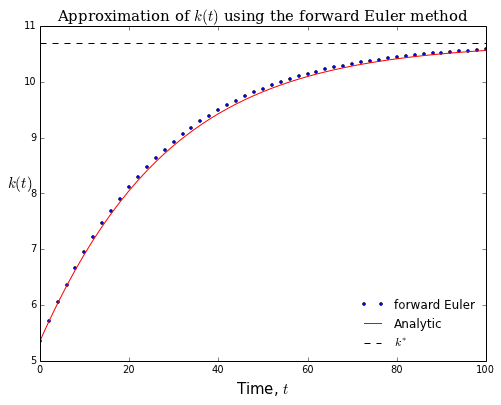This simple method already twice as accurate as linearized solution!

In :
# compute the max approximation error
solow.get_maximal_errors(forward_euler_traj, analytic_trajectory)

Out:
0.067926915448746072

Theory tells us that the approximation error of the forward Euler method is proportional to the step size, $h$. Thus if we decrease the step size by a factor of 10, then the approximation error should also fall by a factor of 10. Let's confirm this result...

In :
# decrease the step size by a factor of 10
forward_euler_traj_2 = solow.integrate(0, k0, h=0.2, T=100, integrator='forward_euler')

# compute new analytic trajectory
grid = forward_euler_traj_2[:,0]
analytic_trajectory_2 = solow_analytic_solution(k0, grid, solow.params)

# compute the max approximation error
solow.get_maximal_errors(forward_euler_traj_2, analytic_trajectory_2)

Out:
0.0066323853348002615

We can also plot the approximation error...

In :
# check out the docstring
solow.plot_approximation_error?

In :
fig = plt.figure(figsize=(8,6))

# plot the approximation errors
ax, traj_error = solow.plot_approximation_error(forward_euler_traj, analytic_trajectory, log=True)
traj_error.set_label('h=2.0')
traj_error.set_marker('o')
traj_error.set_linestyle('none')

ax, traj_2_error = solow.plot_approximation_error(forward_euler_traj_2, analytic_trajectory_2, log=True)
traj_2_error.set_label('h=0.2')
traj_2_error.set_marker('o')
traj_2_error.set_linestyle('none')

# Change the title and add a legend
plt.title('Approximation error using the forward Euler method', fontsize=20, family='serif')
plt.legend(loc='best', frameon=False)

plt.show()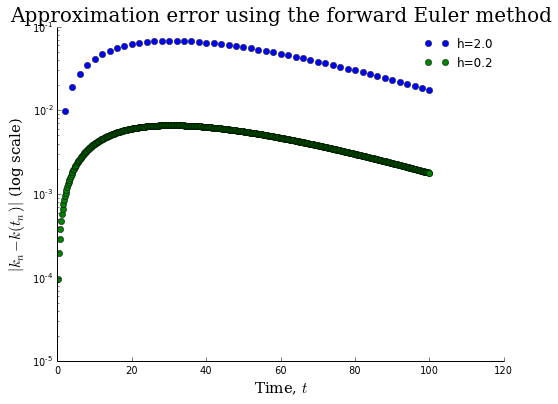### Backward Euler's method¶

The next approximation scheme we are going to look at is called the backward Euler method. Starting from the initial condition, $k_0$, the backward Euler method estimates $k(t_1)$ using the following formula.

$$k_1 = k_0 + h f(t_1, k_1) \tag{6.5}$$

This formula is a non-linear equation in the unknown $k_1$ and defines $k_1$ only implicitly in terms of $t_0, t_1$, and $k_0$ (all of which are given). Repeated application of the above idea yields a recursive formulation of the backward Euler method

$$k_{n+1} = k_n + h f(t_{n+1}, k_{n+1}),\ n=0,1,\dots \tag{6.6}$$

where $h >0$ denotes the size of the step. Note that this method requires solving the same number of non-linear equation as there are grid points $t_n,\ n=0,1,\dots$. Lots of different methods exist for solving non-linear equations (or systems of non-linear equations) and solving non-linear equations continues to be a major research topic in numerical analysis. We will touch on some of these methods (the ones that have proven to be of use in economics research) in more detail later in the course. For now, I just want you to remember that you are solving a system of non-linear equations whenever you use any "implicit" method for solving an IVP.Note that the backward Euler method "undershoots" the solution, $k(t)$, but not quite as badly compared with the forward Euler method. The opposite would be true if the solution had been convex. As was the case with the forward Euler method, the backward Euler method is convergent and the approximation error is proportional to the step size, $h$. In practice, implicit methods like the backward Euler method have proven to be more "stable" (i.e., implicit methods don't always accumulate approximation error as fast as explicit methods) than explicit methods like the forward Euler method and for this reason most "industrial strength" IVP solvers tend to be at least partially implicit.

In :
# solve the model using the backward Euler method
backward_euler_traj = solow.integrate(0, k0, h=2.0, T=100, integrator='backward_euler')

In :
backward_euler_traj

Out:
array([[   0.        ,    5.34659456],
[   2.        ,    5.68732199],
[   4.        ,    6.00945951],
[   6.        ,    6.3134753 ],
[   8.        ,    6.59994302],
[  10.        ,    6.86950953],
[  12.        ,    7.12286994],
[  14.        ,    7.36074818],
[  16.        ,    7.58388198],
[  18.        ,    7.79301129],
[  20.        ,    7.98886939],
[  22.        ,    8.1721761 ],
[  24.        ,    8.34363276],
[  26.        ,    8.50391853],
[  28.        ,    8.65368775],
[  30.        ,    8.79356821],
[  32.        ,    8.92416007],
[  34.        ,    9.04603529],
[  36.        ,    9.15973754],
[  38.        ,    9.26578244],
[  40.        ,    9.36465799],
[  42.        ,    9.45682526],
[  44.        ,    9.54271918],
[  46.        ,    9.6227495 ],
[  48.        ,    9.69730174],
[  50.        ,    9.76673822],
[  52.        ,    9.83139917],
[  54.        ,    9.89160373],
[  56.        ,    9.94765107],
[  58.        ,    9.99982143],
[  60.        ,   10.04837711],
[  62.        ,   10.09356352],
[  64.        ,   10.13561012],
[  66.        ,   10.17473137],
[  68.        ,   10.21112759],
[  70.        ,   10.24498583],
[  72.        ,   10.27648071],
[  74.        ,   10.30577516],
[  76.        ,   10.33302115],
[  78.        ,   10.35836041],
[  80.        ,   10.38192509],
[  82.        ,   10.40383833],
[  84.        ,   10.4242149 ],
[  86.        ,   10.44316173],
[  88.        ,   10.46077843],
[  90.        ,   10.47715775],
[  92.        ,   10.49238607],
[  94.        ,   10.50654381],
[  96.        ,   10.51970585],
[  98.        ,   10.53194186],
[ 100.        ,   10.5433167 ]])
In :
# how fast is the backwards Euler method?
%timeit -n 1 -r 3 solow.integrate(0, 10, 2.0, 1000, integrator='backward_euler')

1 loops, best of 3: 236 ms per loop

In :
# plot the backwards Euler approximation and the analytic solution
plt.figure(figsize=(8,6))

grid = backward_euler_traj[:,0]

plt.plot(grid, backward_euler_traj[:,1], 'go', markersize=3.0, label='backward Euler')
plt.plot(grid, analytic_trajectory[:,1], 'r-', label='Analytic')

# demarcate k*
color='k', label='$k^*$')

# axes, labels, title, etc
plt.xlabel('Time, $t$', fontsize=15)
plt.ylabel('$k(t)$', rotation='horizontal', fontsize=15)
plt.title('Approximation of $k(t)$ using the backward Euler method', fontsize=15)
plt.legend(loc='best', frameon=False)
plt.show()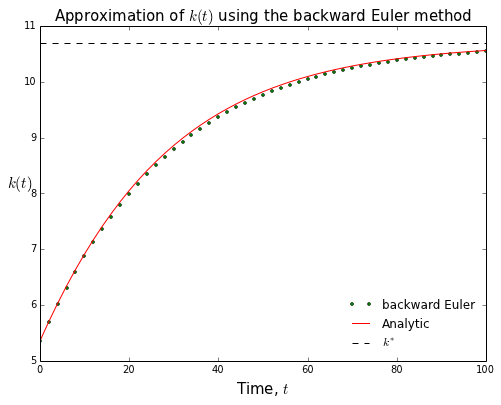In :
# compute the max approximation error...slightly better than the forward Euler method
solow.get_maximal_errors(backward_euler_traj, analytic_trajectory)

Out:
0.064456094680954479

### Trapezoidal rule¶

The Euler method approximates the derivative of the solution by a constant in the interval $[t_n, t_{n+1}]$. Specifically, Euler's method assumes that the slope of the solution in the interval is equal to its value at the left end point, $f(t_n, y_n)$. Intuitively one might guess that a better way to approximate the derivative of the solution by a constant in the interval would be to take a simple average of the slope of the solution at both end points. This simple intuition is formalized by the following approximation scheme

\begin{align} y(t) =& y(t_n) + \int_{t_n}^{t} f(\tau, y(\tau))d\tau \notag \\ \approx& y(t_n) + \frac{1}{2}(t - t_n)f(t_0, y(t_n)) + \frac{1}{2}(t - t_n)f(t_{n+1}, y(t_{n+1})) \tag{6.7} \end{align}

which leads to the trapezoidal rule.

\begin{equation} y_{n+1} = y_n + \frac{1}{2}hf(t_0, y_n) + \frac{1}{2}hf(t_{n+1}, y_{n+1}) \tag{6.8} \end{equation}

Do we know that the trapezoidal rule converges to the exact solution $y(t)$ on the arbitrary interval $[t_0, t_0 + t^*]$ as we shrink the step size $h$ toward zero?. Yes! How fast does the trapezoidal rule converge to the exact solution? The trapezoidal rule can be shown to converge quadratically to the exact solution. This means that unlike the forward and backward Euler methods, which were only linearly convergent, with the trapezoidal rule, shrinking the step size by a factor of 10 will reduce the approximation error by a factor of $10^2$ = 100!The higher order of convergence means that the trapezoidal rule can often make due with a larger step size than the Euler method while still maintaining the same level of approximation error. However, the improved convergence properties of the trapezoidal rule do not come free (remember that there is no such thing as a free lunch!). The trapezoidal method is an implicit method, and each step requires solving a non-linear equation in the unknown $k_{n+1}$ as well as extra evaluation of the function $f$. In practice, however, the more rapid convergence of the trapezoidal method and ability to use a larger step size often more than compensate for the additional computational burden.

In :
# solve the model using the trapezoidal rule
trapezoidal_rule_traj = solow.integrate(0, k0, h=2.0, T=100, integrator='trapezoidal_rule')

In :
trapezoidal_rule_traj

Out:
array([[   0.        ,    5.34659456],
[   2.        ,    5.69651671],
[   4.        ,    6.02716816],
[   6.        ,    6.33895315],
[   8.        ,    6.63241531],
[  10.        ,    6.90819667],
[  12.        ,    7.16700587],
[  14.        ,    7.40959359],
[  16.        ,    7.63673345],
[  18.        ,    7.84920733],
[  20.        ,    8.0477941 ],
[  22.        ,    8.23326101],
[  24.        ,    8.40635729],
[  26.        ,    8.56780944],
[  28.        ,    8.7183178 ],
[  30.        ,    8.85855433],
[  32.        ,    8.9891611 ],
[  34.        ,    9.11074957],
[  36.        ,    9.22390028],
[  38.        ,    9.3291631 ],
[  40.        ,    9.42705761],
[  42.        ,    9.51807388],
[  44.        ,    9.60267335],
[  46.        ,    9.68128984],
[  48.        ,    9.75433066],
[  50.        ,    9.82217778],
[  52.        ,    9.88518903],
[  54.        ,    9.94369932],
[  56.        ,    9.99802178],
[  58.        ,   10.04844905],
[  60.        ,   10.09525433],
[  62.        ,   10.13869257],
[  64.        ,   10.17900155],
[  66.        ,   10.21640289],
[  68.        ,   10.25110307],
[  70.        ,   10.28329441],
[  72.        ,   10.31315589],
[  74.        ,   10.34085412],
[  76.        ,   10.36654406],
[  78.        ,   10.39036982],
[  80.        ,   10.4124654 ],
[  82.        ,   10.43295534],
[  84.        ,   10.45195539],
[  86.        ,   10.46957306],
[  88.        ,   10.48590825],
[  90.        ,   10.50105373],
[  92.        ,   10.51509562],
[  94.        ,   10.52811392],
[  96.        ,   10.54018286],
[  98.        ,   10.55137136],
[ 100.        ,   10.56174336]])
In :
# how fast is the trapezoidal rule
%timeit -n 1 -r 3 solow.integrate(0, 10, h=2.0, T=1000, integrator='trapezoidal_rule')

1 loops, best of 3: 304 ms per loop

In :
# plot the trapezoidal rule approximation and the analytic solution
plt.figure(figsize=(8,6))

grid = trapezoidal_rule_traj[:,0]

plt.plot(grid, trapezoidal_rule_traj[:,1], 'yo', markersize=3.0, label='trapezoidal rule')
plt.plot(grid, solow_analytic_solution(k0, grid, solow.params)[:,1], 'r-', label='Analytic')

# demarcate k*
color='k', label='$k^*$')

# axes, labels, title, etc
plt.xlabel('Time, $t$', fontsize=15)
plt.ylabel('$k(t)$', rotation='horizontal', fontsize=15)
plt.title('Approximation of $k(t)$ using the trapezoidal rule', fontsize=15)
plt.legend(loc='best', frameon=False)
plt.show()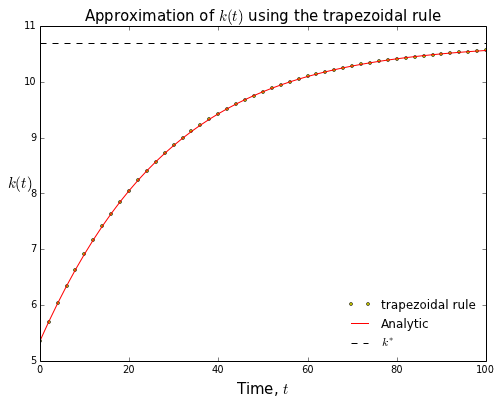In :
# compute the max approximation error for the trapezoidal rule
solow.get_maximal_errors(trapezoidal_rule_traj, analytic_trajectory)

Out:
0.00056222209814471569
In :
# decrease the step size by a factor of 10...
trapezoidal_rule_traj_2 = solow.integrate(0, k0, h=0.2, T=100, integrator='trapezoidal_rule')

In :
# compute the max approximation error for the trapezoidal rule
solow.get_maximal_errors(trapezoidal_rule_traj_2, analytic_trajectory_2)

Out:
5.6228340188368975e-06
In :
# plot the approximation error
fig = plt.figure(figsize=(8,6))

# plot the approximation errors
ax, traj_error = solow.plot_approximation_error(trapezoidal_rule_traj, analytic_trajectory, log=True)
traj_error.set_label('h=2.0')
traj_error.set_marker('o')
traj_error.set_linestyle('none')

ax, traj_2_error = solow.plot_approximation_error(trapezoidal_rule_traj_2, analytic_trajectory_2, log=True)
traj_2_error.set_label('h=0.2')
traj_2_error.set_marker('o')
traj_2_error.set_linestyle('none')

# Change the title and add a legend
plt.title('Approximation error using the trapezoidal rule', fontsize=20, family='serif')
plt.legend(loc='best', frameon=False)

plt.show()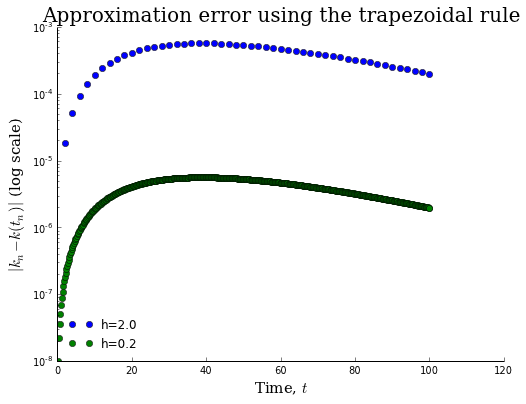## Task 7: Piece-wise Linear Interpolation¶

The above approximation schemes all discretize the independent variable, $t$, and approximate the function $k(t)$ at only a finite number of grid points. What if we need to approximate the value of $k(t)$ in between grid points? Approximating the values of a function between grid points is called interpolation (interpolation happens to be a special case of another technique with which you are already very familiar...namely regression!).

The specific interpolation routine that we will use is called piece-wise linear interpolation. Linear interpolation is the most basic a method of approximating the value of a function between two points. When you connect two dots with a straight line you are doing linear interpolation. Formally, linear interpolation approximates the function $k$ on the interval $t\in[t_n, t_{n+1}]$ as

$$k(t) \approx k_n + \frac{t - t_n}{t_{n+1} - t_n}(k_{n+1} - k_n). \tag{7.1}$$

Here is a nice graphic that gives the basic intuition linear interpolation...Linear interpolation is a simple, yet robust method, and it will usually be a good place to start when doing your own research projects.

In [ ]:
# always check the docstring!
solow.interpolate?

In :
# create some grid of values for t
grid = np.linspace(0, 100, 1000)

# simulate a trajectory using linear interpolation to fill in the values between nodes
interp_trapezoidal_rule_traj = solow.interpolate(traj=trapezoidal_rule_traj, ti=grid, k=1)

# compute new analytic trajectory
analytic_trajectory_3 = solow_analytic_solution(k0=k0, t=grid, params=solow.params)

In :
# plot the trapezoidal rule approximation and the analytic solution
plt.figure(figsize=(8,6))

plt.plot(grid, interp_trapezoidal_rule_traj[:,1], 'y', markersize=3.0, label='Trapezoidal rule (linear)')
plt.plot(grid, analytic_trajectory_3[:,1], 'r-', label='Analytic')

# demarcate k*
color='k', label='$k^*$')

# axes, labels, title, etc
plt.xlabel('Time, $t$', fontsize=15)
plt.ylabel('$k(t)$', rotation='horizontal', fontsize=15)
plt.title('Approximating $k(t)$ using the trapezoidal rule, redux', fontsize=20, family='serif')
plt.legend(loc='best', frameon=False)
plt.show()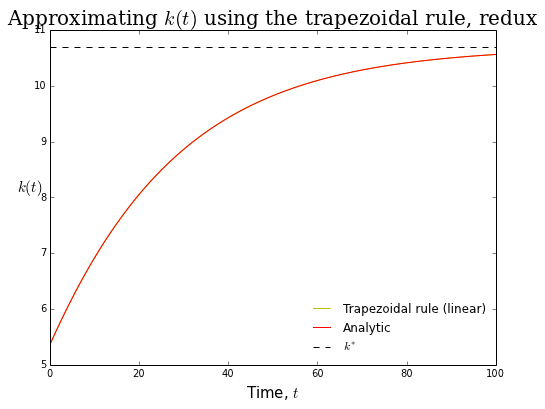In :
# plot the approximation error
fig = plt.figure(figsize=(8,6))

# plot the approximation errors
ax, traj_error = solow.plot_approximation_error(interp_trapezoidal_rule_traj, analytic_trajectory_3, log=True)
traj_error.set_label('h=2.0')

# Change the title and add a legend
plt.title('Trapezoidal rule with linear interpolation', fontsize=20, family='serif')
plt.legend(loc='best', frameon=False)

plt.show()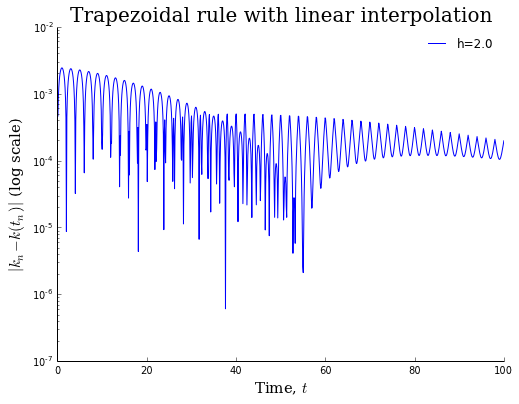None of the approximation schemes that we discussed today are robust enough to use in your own research. However all of the more sophisticated solvers are based on the same principles used in the approaches detailed above and in your notes. The point of this task was not to expose you to state of the art methods, but rather to give you some intuition as to how the more robust and sophisticated methods work.

Four of the best, most widely used ODE integrators have been implemented in the scipy.integrate module (they are called dopri5, dop85, lsoda, and vode). Each of these integrators uses some type of adaptive step-size control: the integrator adaptively adjusts the step size $h$ in order to keep the approximation error below some user-specified threshold). The cells below contain code which compares the approximation error of the forward Euler with those of lsoda and vode. Instead of simple linear interpolation (i.e., k=1), I set k=5 for 5th order B-spline interpolation.

In :
# grid of interpolatiom points
interp_grid = np.linspace(0, 200, 1000)

# solve using the lsoda integrator
kwargs={'with_jacobian':True, 'rtol':1e-9}
lsoda_traj = solow.integrate(0, k0, 1.0, T=200, integrator='lsoda', **kwargs)
lsoda_traj_interp = solow.interpolate(lsoda_traj, interp_grid, k=5)

# solve using the vode integrator
vode_traj = solow.integrate(0, k0, 1.0, T=200, integrator='vode', **kwargs)
vode_traj_interp = solow.interpolate(vode_traj, interp_grid, k=5)

# solve using the trapezoidal rule
am1_traj = solow.integrate(0, k0, 1.0, T=200, integrator='backward_euler')
am1_traj_interp = solow.interpolate(am1_traj, interp_grid, k=5)

# compute the analytic trajectory
grid = lsoda_traj[:,0]
analytic_trajectory = solow_analytic_solution(k0, interp_grid, solow.params)

# compute the linearized trajectory
linearized_trajectory = solow.linearized_solution(k0, interp_grid)

In :
fig = plt.figure(figsize=(8,6))
colors = mpl.cm.jet(np.linspace(0, 1, 4))

# plot the approximation error for lsoda
traj_error = solow.plot_approximation_error(lsoda_traj_interp,
analytic_trajectory,
log=True)
traj_error.set_label('lsoda')
traj_error.set_color(colors)

# plot the approximation error for vode
traj_error_2 = solow.plot_approximation_error(vode_traj_interp,
analytic_trajectory,
log=True)
traj_error_2.set_label('vode')
traj_error_2.set_color(colors)

# plot the approximation error for trapezoidal rule
traj_error_3 = solow.plot_approximation_error(am1_traj_interp,
analytic_trajectory,
log=True)
traj_error_3.set_label('Backward Euler')
traj_error_3.set_color(colors)

# plot the approximation error for linearized trajectory
traj_error_4 = solow.plot_approximation_error(linearized_trajectory,
analytic_trajectory,
log=True)
traj_error_4.set_label('Linearization')
traj_error_4.set_color(colors)

# demarcate machine eps
plt.axhline(np.finfo('float').eps, color='k', ls='--',
label=r'Machine-$\epsilon$')

# change the title and add a legend
plt.ylim(1e-18, 1e0)
plt.title('The benefits of using adaptive step-size control\n' +
'with linear multi-step methods', fontsize=20, family='serif')
plt.legend(loc='best', frameon=False, prop={'family':'serif'}, bbox_to_anchor=(1.025, 1.0))
plt.show()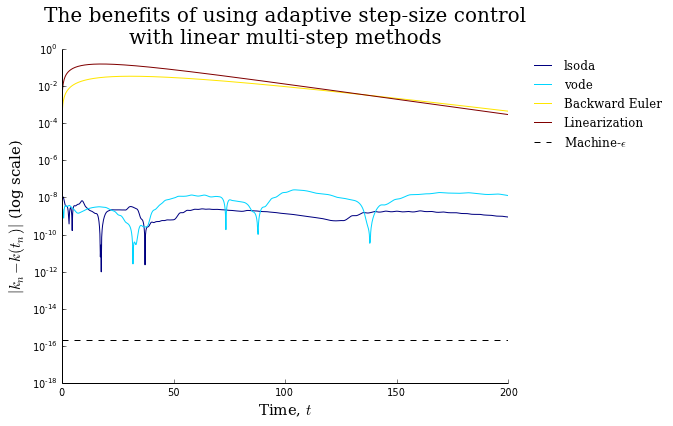In [ ]: